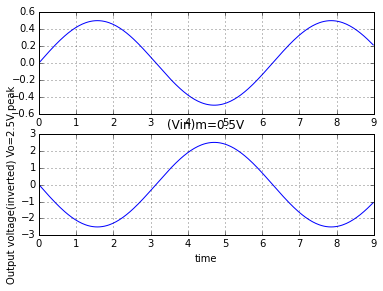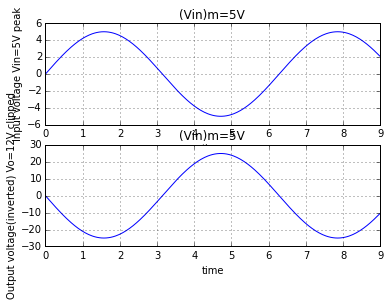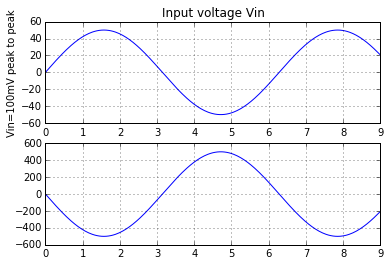# Chapter 10 Introduction to operational amplifiers¶

## Ex 10_1 PG-10.7¶

In :
from __future__ import division
print "Refer to the figure-10.5 shown"
V1=300e-6#
V2=240e-6#
Vd=V1-V2#differential mode voltage
Vc=(V1+V2)/2##common mode voltage
print "\n when CMRR=100"
CMRR=100##common mode rejection ratio
print "\n common mode gain Ac=%.f \n"%(Ac)
print " output voltage is Vo=%.1f mV \n"%(Vo*1e3)
print "\n when CMRR=100000"
CMRR=1e5##common mode rejection ratio
print "\n common mode gain Ac=%.2f \n"%(Ac)
print " output voltage is Vo=%.4f mV \n"%(Vo*1e3)

Refer to the figure-10.5 shown

when CMRR=100

common mode gain Ac=50

output voltage is Vo=313.5 mV

when CMRR=100000

common mode gain Ac=0.05

output voltage is Vo=300.0135 mV



## Ex 10_2 PG-10.17¶

In :
Ib1=18##in microA
Ib2=22##in microA
Ib=(Ib1+Ib2)/2##input bias current
Ib=Ib
print "\n input bias current= %.0f microA \n"%(Ib)
Iios=(Ib1-Ib2)##input offset current
Iios=abs(Iios)#
Iios=Iios
print "\n input offset current= %.0f microA \n"%(Iios)

 input bias current= 20 microA

input offset current= 4 microA



## Ex 10_3 PG-10.17¶

In :
import numpy as np
Iios=20##Input offset current in nA
Ib=60##Input bias current in nA
#Iios=Ib1-Ib2=20
#Ib=(Ib1+Ib2)/2=60
#ie Ib=(Ib1+Ib2)=120
print " Iios=Ib1-Ib2=20"
print " ie Ib=(Ib1+Ib2)/2=60 ie Ib=(Ib1+Ib2)=120"
a=np.mat([[1 ,-1],[1, 1]])##coefficient of Ib1 and Ib2 for Iios and Ib
b=np.mat([, ])##value of Iios and Ib
x=(a*-1)*b
print " values of Ib1 andIb2 are"
print "  %.0f & %0.0f nA "%(x[0,0],x[1,0])

 Iios=Ib1-Ib2=20
ie Ib=(Ib1+Ib2)/2=60 ie Ib=(Ib1+Ib2)=120
values of Ib1 andIb2 are
100 & -140 nA


## Ex 10_4 PG-10.35¶

In :
print "Refer to the figure-10.32 shown"
#the circuit is an inverting amplifier
R1=10e3#
Rf=47e3##feedback resistance
A=-Rf/R1##gain of an inverting amplifier
print "\n the gain is %.1f (inverting amplifier) \n"%(A)

Refer to the figure-10.32 shown

the gain is -4.7 (inverting amplifier)



## Ex 10_5 PG-10.35¶

In :
from numpy import pi, sin
import numpy as np
%matplotlib inline
from matplotlib import pyplot as plt

R1=10e3#
Rf=50e3##feedback resistance
Vcc=12##supply voltage in volts
A=-Rf/R1##gain of an inverting amplifier
A=abs(A)##magnitude of the gain
print "If Vm=0.5V then"
Vm=0.5##peak value of the input voltage
Vo=A*Vm##output voltage
print "\n peak value of the output voltage Vo=%.1f V \n"%(Vo)
print "\n if Vm=5V then"
Vm=5##peak value of the input voltage
Vo1=A*Vm##output voltage
print "\n peak value of the output voltage Vo=%.0f V \n"%(Vo1)
print "but the opamp output saturates at +/- 12V hence portion above +12V and"
print " below -12V will be clipped off.So 25V \n peak output is not practically possible it will show upto +/- 12V"
plt.subplot(2,1,1)        #input voltage Vin=0.5V peak
x=np.arange(0,9,pi/100)
y=0.5*sin(x)
plt.plot(x,y)
#plt.xtitle("(Vin)m=0.5V" ,"time"%("Input voltage Vin= 0.5V peak"
plt.grid()
plt.subplot(2,1,2)         #output voltage Vo=2.5V peak
x=np.arange(0,9,pi/100)
y=-Vo*sin(x)        #output is inverted
plt.plot(x,y)
plt.title("(Vin)m=0.5V" )
plt.xlabel("time")
plt.ylabel("Output voltage(inverted) Vo=2.5V peak")
plt.grid()
plt.show()

plt.subplot(2,1,1)        #input voltage Vin=5V peak
x=np.arange(0,9,pi/100)
y=5*sin(x)
plt.plot(x,y)
plt.title("(Vin)m=5V" )
plt.xlabel("time")
plt.ylabel("Input voltage Vin=5V peak")
plt.grid()
plt.subplot(2,1,2)        #output voltage Vo=25V peak but clipped at + or-12V
x=np.arange(0,9,pi/100)
y=-Vo1*sin(x)       #output is inverted
#y(find(y > 12)) =  12#
#y(find(y < -12))= -12#
plt.plot(x,y)
plt.title("(Vin)m=5V")
plt.xlabel("time")
plt.ylabel("Output voltage(inverted) Vo=12V clipped")
plt.grid()
plt.show()

If Vm=0.5V then

peak value of the output voltage Vo=2.5 V

if Vm=5V then

peak value of the output voltage Vo=25 V

but the opamp output saturates at +/- 12V hence portion above +12V and
below -12V will be clipped off.So 25V
peak output is not practically possible it will show upto +/- 12V## Ex 10_6 PG-10.36¶

In :
from numpy import sqrt
R1=10e3#
Rf=47e3##feedback resistance
Vcc=12##supply voltage
A=-Rf/R1##gain of an inverting amplifier
A=abs(A)##magnitude of the gain of an inverting amplifier
Vin=2##peak to peak input voltage
Voo=Vin*A##peak to peak output voltage
#the AC voltmeter measures the rms value
Vo=Voo/2##peak output voltage
Vrms=Vo/sqrt(2)##rms value of the output voltage
print "\n Reading on the AC voltmeter is %.4f V \n"%(Vrms)

 Reading on the AC voltmeter is 3.3234 V



## Ex 10_7 PG-10.37¶

In :
%matplotlib inline
from matplotlib.pyplot import plot, subplot, title, xlabel, ylabel, show, grid
subplot(2,1,1)    #input voltage Vin=0.5V peak
from numpy import arange, pi, sqrt, sin

R1=1e3#
Rf=10e3##feedback resistance
Vin=100e-3##input voltage peak to peak
A=-Rf/R1##gain of an inverting amplifier
Vo=A*Vin#
print "\n peak to peak value of the output voltage Vo = %.0f V \n"%(Vo)
print "\n voltage gain of the inverting amplifier  Af = %.0f \n "%(A)
#plotting of the waveforms
x=arange(0,9,pi/100)
y=50*sin(x)
plot(x,y)
title("Input voltage Vin ")
xlabel("time")
ylabel("Vin=100mV peak to peak")
grid()
subplot(2,1,2)      #output voltage Vo=2.5V peak
x=arange(0,9,pi/100)
y=-500*sin(x)
plot(x,y)
#xtitle("Output voltage Vo" ,"time"%("Vo=1000mV peak to peak"
grid()

 peak to peak value of the output voltage Vo = -1 V

voltage gain of the inverting amplifier  Af = -10## Ex 10_8 PG-10.40¶

In :
A=61##gain required for the non inverting amplifier
R1=1e3#
print "Refer to the figure-10.36 shown\n"
print " \n The gain of the non inverting amplifier is A=1+Rf/R1"
#the gain of the non inverting amplifier is A=1+Rf/R1
x=A-1##x=Rf/R1
Rf=x*R1#
print "\n\n Therefore feedback resistance Rf=%.0f kohm \n"%(Rf*1e-3)

Refer to the figure-10.36 shown

The gain of the non inverting amplifier is A=1+Rf/R1

Therefore feedback resistance Rf=60 kohm



## Ex 10_9 PG-10.40¶

In :
R1=1e3#
Rf=10e3##feedback resistance
A=1+Rf/R1##gain of a non-inverting amplifier
print "Gain is %.0f\n"%(A)
print "For Vin =0.5V "
Vin=0.5##input voltage
Vo=A*Vin#
print " Output voltage Vo=%.1f V \n"%(Vo)
print "For Vin =-3V "
Vin=-3##input voltage
Vo=A*Vin#
print " Output voltage Vo=%.1f V \n"%(Vo)
print "\n but Vo=-33V is not possible. Output will saturate at -12V \n"
print " And the remaining portion will be clipped from output."

Gain is 11

For Vin =0.5V
Output voltage Vo=5.5 V

For Vin =-3V
Output voltage Vo=-33.0 V

but Vo=-33V is not possible. Output will saturate at -12V

And the remaining portion will be clipped from output.


## Ex 10_10 PG-10.48¶

In :
print "Refer to the figure-10.43 shown\n "
#A is grounded so B is virtual ground
#Vb=Va=0
Vb=0#
R1=1e3#
R2=5e3#
R3=5e3#
R4=100#
print "\n Vb=Va=0  ................(1)"
print "\n Vb=0 \n I1=(Vin-Vb)/R1=Vin/R1 \n I1=(Vb-Vx)/R2=-Vx/R2 \n \n   Vin/Rf=-Vx/R2"
print "\n =>Vx=-R2/R1*Vin........(2) \n \n now Vx=I2*R4 and (I1-I2)=(Vx-Vo)/R3 \n"
print " =>I2=Vx/R4 and I1-Vx/R4=(Vx-Vo)/R3 \n Therefore \n Vin/R1-Vx/R4=(Vx-Vo)/R3 ...."
print ".......using I1=Vin/R1 \n Vin/R1-Vx(1/R4+1/R3)=-Vo/R3 \n \n "
print "Vin/R1-(-R2/R1)*Vin*(1/R4+1/R3)=-Vo/R3 ........using (2) \n"
print " Vin*(1/R1+R2/R1*(1/R4+1/R3))=-Vo/R3 \n \n "
print "Vin=-(R1*R4/(R3*R4+R2*R3+R2*R4))*Vo \n \n"
print " Acl=Vo/Vin=-(R3*R4+R2*R3+R2*R4)/(R1*R4) \n"
Acl=-(R3*R4+R2*R3+R2*R4)/(R1*R4)#
print "\n closed loop gain Acl=%.0f \n"%(Acl)
Acl=abs(Acl)#
Rf=R1*Acl##equivalent feedback resistance
print "\n equivalent feedback resistance Rf= %.0f kohm "%(Rf*1e-3)

Refer to the figure-10.43 shown

Vb=Va=0  ................(1)

Vb=0
I1=(Vin-Vb)/R1=Vin/R1
I1=(Vb-Vx)/R2=-Vx/R2

Vin/Rf=-Vx/R2

=>Vx=-R2/R1*Vin........(2)

now Vx=I2*R4 and (I1-I2)=(Vx-Vo)/R3

=>I2=Vx/R4 and I1-Vx/R4=(Vx-Vo)/R3
Therefore
Vin/R1-Vx/R4=(Vx-Vo)/R3 ....
.......using I1=Vin/R1
Vin/R1-Vx(1/R4+1/R3)=-Vo/R3

Vin/R1-(-R2/R1)*Vin*(1/R4+1/R3)=-Vo/R3 ........using (2)

Vin*(1/R1+R2/R1*(1/R4+1/R3))=-Vo/R3

Vin=-(R1*R4/(R3*R4+R2*R3+R2*R4))*Vo

Acl=Vo/Vin=-(R3*R4+R2*R3+R2*R4)/(R1*R4)

closed loop gain Acl=-260

equivalent feedback resistance Rf= 260 kohm


## Ex 10_11 PG-10.50¶

In :
print "Refer to the figure-10.45 and figure-10.45(a)shown"
#the circuit is a non inverting amplifier
Vin=10##input voltage
#opamp input current is zero...
R1=10e3##resistance connected to the -ve terminal of the amplifier
R2=1e3##resistance connected to the +ve terminal of the amplifier to the input voltage
R3=1e3###resistance connected to the +ve terminal of the amplifier to the gound
Rf=50e3##feedback resistance
I=Vin/(R2+R3)#
Vb=I*R3#
V0=(1+Rf/R1)*Vb##output voltage
print "\n output voltage Vo=%.f V \n"%(V0)

Refer to the figure-10.45 and figure-10.45(a)shown

output voltage Vo=30 V



## Ex 10_12 PG-10.51¶

In :
print "Refer to the figure-10.46 and figure-10.46(a)shown"
print "We split the circuit of the figure-10.46 as shown in figure-10.46(a)"
print "For first stage the circuit is a non-inverting amplifier"
Rf=10e3##feedback resistance for the first stage of the circuit
R1=100e3##value of R1 for the first stage of the circuit
from sympy import symbols
V1=symbols('V1')##V1=Vin
Vo1=(1+Rf/R1)*V1#
print "Therefore Vo1 =",Vo1

print "\n For the second stage we use superposition principle.\n We use each input at one time\n"
print "First we assume Vo1 is active and V2 is grounded as shown in figure10.46(b)"
Rf1=100e3##feedback resistance for figure-10.46(b)
R11=10e3##value of R1 for figure1-10.46(a)
Vo_=-Rf1/R11*Vo1##when V2=0V as shown in figure-10.46(b)
print "Therefore Vo_ =",Vo_

print "then we assume V2 is active and Vo1 is grounded as shown in figure10.46(c)"
V2=symbols('V2')##V2=Vin
Vo__=(1+Rf1/R11)*V2##when Vo1=0V as shown in figure-10.46(c),it is a non inverting amplifier
print "Therefore Vo__ =",Vo__
print "\n   Therefore output voltage Vo = Vo_ + Vo__= 11V2-11V1 "
print "  =>Vo=11(V2-V1)"

Refer to the figure-10.46 and figure-10.46(a)shown
We split the circuit of the figure-10.46 as shown in figure-10.46(a)
For first stage the circuit is a non-inverting amplifier
Therefore Vo1 = 1.1*V1

For the second stage we use superposition principle.
We use each input at one time

First we assume Vo1 is active and V2 is grounded as shown in figure10.46(b)
Therefore Vo_ = -11.0*V1
then we assume V2 is active and Vo1 is grounded as shown in figure10.46(c)
Therefore Vo__ = 11.0*V2

Therefore output voltage Vo = Vo_ + Vo__= 11V2-11V1
=>Vo=11(V2-V1)


## Ex 10_13 PG-10.62¶

In :
print "Refer to the figure-10.55 and shown"
R1=1e3#
Rf=1e3##feedback resistance
Vin1=2#
Vin2=1#
Vin3=4#
Vout=-(Vin1+Vin2+Vin3)*Rf/R1
print "\n output voltage Vout=%.f V \n"%(Vout)

Refer to the figure-10.55 and shown

output voltage Vout=-7 V



## Ex 10_14 PG-10.62¶

In :
print "\n   Vo = -(3V1 + 4V2 + 5V3)\n\n"
Rf=120##we assume feedback resistance to be equal to 120kohm
R1=Rf/3##Rf/R1=3 given
R2=Rf/4##Rf/R2=4 given
R3=Rf/5##Rf/R3=5 given
print "   R1= %.0fkohm  R2= %.0fkohm R3 = %.0fkohm\n"%(R1,R2,R3)
print "\n   The circuit design is shown"

   Vo = -(3V1 + 4V2 + 5V3)

R1= 40kohm  R2= 30kohm R3 = 24kohm

The circuit design is shown


## Ex 10_16 PG-10.64¶

In :
## print "Refer to the figure-10.58 and figure-10.58(a)shown"
R1=1e3#
R2=R1#
Rf=5e3##feedback resistance
R=1e3##resistance connected to the inverting terminal
V1=1##first input voltage at the non inverting terminal
V2=3##second input voltage at the non inverting terminal
Vb=(V1*R2+V2*R1)/(R1+R2)##voltage at the non inverting terminal
Vo=(1+Rf/R)*Vb##output voltage
print "\n Therefore output voltage is Vo=%.0f V \n"%(Vo)
#alternatively we can find the output voltage by the following equation
Vo1=(R2*(R+Rf))/(R*(R1+R2))*V1+(R1*(R+Rf))/(R*(R1+R2))*V2
print "\n Vo=%.0f \n"%(Vo1)

 Therefore output voltage is Vo=12 V

Vo=12



## Ex 10_17 PG-10.65¶

In :
print "Refer to the figure-10.59 shown"
Rf=10e3##feedback resistance
R1=10e3#
R2=20e3#
R3=30e3#
R4=40e3#
V1=-1##first input voltage at the inverting terminal
V2=2##second input voltage at the  inverting terminal
V3=3##third input voltage at the inverting terminal
V4=-2##fourth input voltage at the inverting terminal
Vo=-(Rf/R1*V1+Rf/R2*V2+Rf/R3*V3+Rf/R4*V4)##output voltage
print "\n Therefore output voltage is Vo=%.1f V \n"%(Vo)

Refer to the figure-10.59 shown

Therefore output voltage is Vo=-0.5 V



## Ex 10_18 PG-10.66¶

In :
Rf=1e6##feedback resistance
R1=200e3#
R2=250e3#
R3=500e3#
V1=-2##first input voltage at the inverting terminal
V2=2##second input voltage at the  inverting terminal
V3=11##third input voltage at the inverting terminal
Vo=-(Rf/R1*V1+Rf/R2*V2+Rf/R3*V3)##output voltage
print "\n Therefore output voltage is Vo=%.0f V \n"%(Vo)
#in the book the output Vo=-20V if the value of V3=11V
#but in the question the value of V3=1V so
#I have taken V3=11V so that the Vo=-20V

 Therefore output voltage is Vo=-20 V



## Ex 10_19 PG-10.66¶

In :
Rf=60e3##feedback resistance
R1=10e3#
R2=20e3#
R3=30e3#
V1=-1##first input voltage at the inverting terminal
V2=-2##second input voltage at the  inverting terminal
V3=3##third input voltage at the inverting terminal
Vo=-(Rf/R1*V1+Rf/R2*V2+Rf/R3*V3)##output voltage
print "\n Therefore output voltage is Vo= %.0f V \n"%(Vo)

 Therefore output voltage is Vo= 6 V



## Ex 10_20 PG-10.67¶

In :
from __future__ import division
print "\n   Vo = -(0.1V1 + 0.5V2 + 20V3)\n\n"
Rf=10##we assume feedback resistance to be equal to 10kohm
R1=Rf/0.1##Rf/R1=0.1 given
R2=Rf/0.5##Rf/R2=0.5 given
R3=Rf/20##Rf/R3=20 given
print "   R1= %.0f kohm  R2= %.0f kohm R3 = %.0f ohm\n"%(R1,R2,R3*1e3)
print "\n   The circuit design is shown"

   Vo = -(0.1V1 + 0.5V2 + 20V3)

R1= 100 kohm  R2= 20 kohm R3 = 500 ohm

The circuit design is shown


## Ex 10_21 PG-10.70¶

In :
from __future__ import division
print "\n   Vo = (2V1 - 3V2 + 4V3 - 5V4)\n\n"
print "   The positive terms and negative terms can be added separately\n\n"
print "\n   Vo1 = (2V1 + 4V3 )\n"
Rf1=100##we assume feedback resistance to be equal to 100kohm
R1=Rf1/2##Rf/R1=2 given
R3=Rf1/4##Rf/R3=4 given
print "   Therefore R1= %.0fkohm   R3 = %.0fkohm\n"%(R1,R3)

print "\n   Vo2 = -(3V2 + 5V4)\n"
Rf2=120##we assume feedback resistance to be equal to 120kohm
R2=Rf2/3##Rf/R2=3 given
R4=Rf2/5##Rf/R4=5 given
print "   Therefore R2= %.0fkohm   R4 = %.0fkohm\n"%(R2,R4)
print "\n   The output voltage is Vo = Vo2-Vo1 = (2V1 - 3V2 + 4V3 - 5V4)"
print "\n   The circuit design is shown"
print "\n   For the subtractor we use R = 100kohm  "

   Vo = (2V1 - 3V2 + 4V3 - 5V4)

The positive terms and negative terms can be added separately

Vo1 = (2V1 + 4V3 )

Therefore R1= 50kohm   R3 = 25kohm

Vo2 = -(3V2 + 5V4)

Therefore R2= 40kohm   R4 = 24kohm

The output voltage is Vo = Vo2-Vo1 = (2V1 - 3V2 + 4V3 - 5V4)

The circuit design is shown

For the subtractor we use R = 100kohm


## Ex 10_22 PG-10.76¶

In :
from __future__ import division
R1=100e3#
Cf=1e-6#
Vm=6e-3##peak value of the input voltage
fr=2e3##frequency  supplied at the input
a=-Vm/(R1*Cf)#constant
print "\n   We integrate Vin ie Vo = %.2f*integrate(sin4*pi*100)\n\n"%(a)
def f(x):
y=sin(w*x)
return y
t=input('Enter the value of t (between 0 and 1) :  ')
#the limit is from 0 to t

   We integrate Vin ie Vo = -0.06*integrate(sin4*pi*100)